Home > Industry Information > Analysis of several common diode rectifier circuits, waveform analysis of thyristor rectifier circuit
Analysis of several common diode rectifier circuits, waveform analysis of thyristor rectifier circuit

# Analysis of several common diode rectifier circuits, waveform analysis of thyristor rectifier circuit

2019-04-16

Common diode rectification circuit analysis:

The diode half-wave rectification circuit actually utilizes the unidirectional conduction characteristics of the diode.

When the input voltage is in the positive half cycle of the AC voltage, the diode is turned on and the output voltage vo=vi-vd. When the input voltage is in the negative half cycle of the AC voltage, the diode is turned off and the output voltage vo=0. The waveforms of the input and output voltages of the half-wave rectifier circuit are shown in the figure.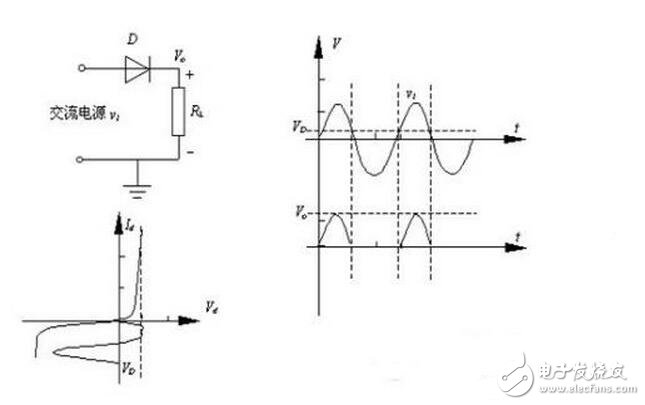For power-type electrical equipment such as motors that use a DC power supply, the ripple voltage of the half-wave rectified output is sufficient. However, for electronic circuits, this voltage cannot be directly used as a power source for semiconductor devices, and must be smoothed (filtered). The smoothing circuit actually connects a capacitor at the output of the half-wave rectification. When the AC voltage is positive for half a cycle, the AC power source charges the capacitor while supplying power to the load through the diode. When the AC voltage is negative for half a week, the capacitor passes through the load resistor. Discharge.Through the above analysis, the basic characteristics of the half-wave rectifier circuit can be obtained as follows:

(1) The half-wave rectified output is a DC ripple voltage.

(2) The AC utilization rate of the half-wave rectifier circuit is 50%.

(3) In the capacitor output half-wave rectification circuit, the diode bears the maximum reverse voltage of 2 times the AC peak voltage (the voltage is superimposed when the capacitor is output).

(3) In the actual circuit, the selection of the half-wave rectifier circuit diode and capacitor must meet the load current requirements.

Full wave rectification

When the input voltage is in the positive half cycle of the AC voltage, the diode D1 is turned on, and the output voltage Vo=vi-VD1. When the input voltage is in the negative half cycle of the AC voltage, the diode D2 is turned on, and the output voltage Vo=vi-VD2.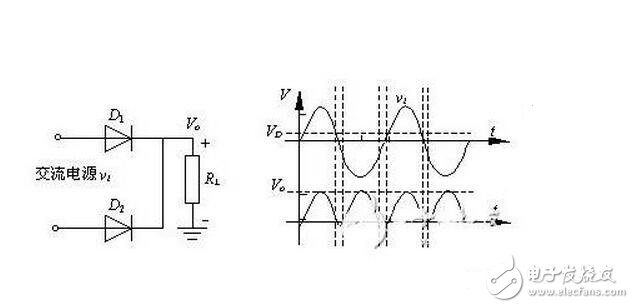It can be seen from the above analysis that the diode full-wave rectification circuit still outputs a pulsating voltage with a constant direction, but the pulsation frequency is twice that of the half-wave rectification.

Various rectifier circuits composed of crystal diodes.

One, half wave rectifier circuit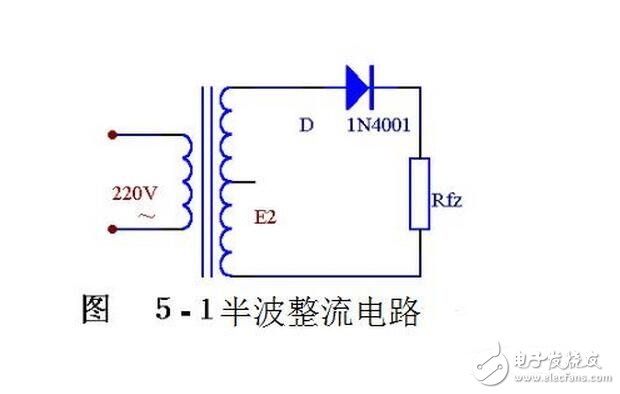Let's see how the diode is rectified from the waveform diagram in Figure 5-2. Figure 5-1 shows the simplest rectifier circuit. It consists of a power transformer B, a Rectifier Diode D and a load resistor Rfz. The transformer converts the mains voltage (mostly 220 volts) into the required alternating voltage e2, which in turn converts the alternating current into pulsating direct current.

The transformer cut-off voltage e2 is a sine wave voltage whose direction and magnitude change with time. Its waveform is shown in Figure 5-2(a). In the 0~K time, e2 is positive half cycle, that is, the upper end of the transformer is negative at the lower end. At this time, the diode is subjected to the forward voltage surface conduction, and e2 is applied to the load resistor Rfz through it. In the period of π~2π, e2 is a negative half cycle, and the lower end of the transformer is positive and the upper end is negative. At this time, D is subjected to reverse voltage, non-conducting, and there is no voltage on Rfz. In the π~2π time, the process of 0~π time is repeated, and in the 3π~4π time, the process of π~2π time is repeated... This way, the negative half cycle of the alternating current is "cut", only positive Half-cycle through Rfz, a single right-direction (upper-down-down) voltage is obtained on Rfz, as shown in Figure 5-2(b), the rectification purpose is achieved, but the load voltage Usc. And the magnitude of the load current also varies with time, so it is often referred to as pulsating DC.This rectification method of removing the half cycle and the lower half of the figure is called half-wave rectification. It is not difficult to see that the half-wave symmetry is exchanged for the rectification effect at the expense of "sacrifice" half of the AC, and the current utilization rate is very low (calculation shows that the average value of the half-wave voltage obtained by rectification in the whole cycle, that is, the load The DC voltage Usc = 0.45e2) is therefore commonly used in high voltage, low current applications, and is rarely used in general radios.

Diode rectifier circuit circuit Appreciation Full-wave rectifier circuit

If the structure of the rectifier circuit is adjusted, a full-wave rectifier circuit that can fully utilize the power can be obtained. Figure 5-3 shows the electrical schematic of a full-wave rectifier circuit.The full-wave rectification circuit can be considered as a combination of two half-wave rectification circuits. In the middle of the secondary winding of the transformer, a tap is drawn to divide the sub-group coil into two symmetrical windings, thereby extracting two equal-sized but opposite-polarized voltages e2a, e2b, which constitute e2a, D1, Rfz and e2b, D2, Rfz, Two energized circuits.

The working principle of the full-wave rectifier circuit can be illustrated by the waveform diagram shown in Figure 5-4. In the range of 0~π, e2a is a forward voltage to D1, D1 is turned on, and a positive and negative voltage is obtained on Rfz; e2b is a reverse voltage for D2, and D2 is not conducting (see Figure 5-4 (b) In π-2π time, e2b is forward voltage for D2, D2 is turned on, and the voltage obtained on Rfz is still positive and negative; e2a is reverse voltage for D1, and D1 is not conductive (see Figure 5-4 (C).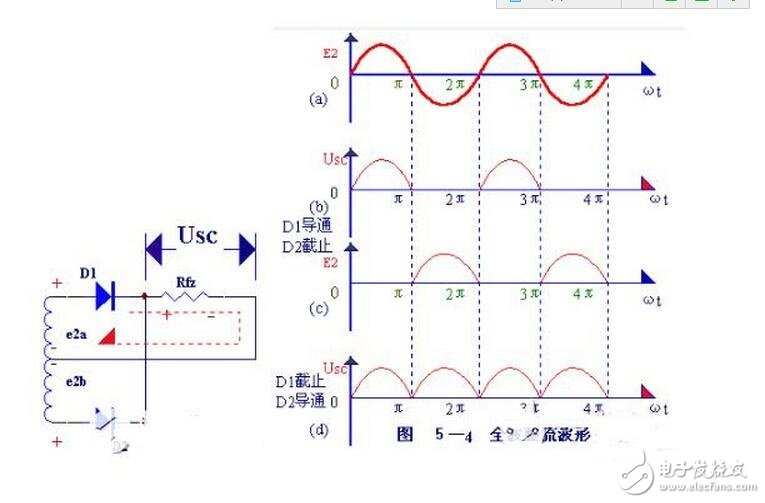In this way, since the two rectifying elements D1 and D2 are alternately conducting, the load resistance Rfz has a current in the same direction during the positive and negative two half cycles, as shown in FIG. 5-4(b). Therefore, it is called full-wave rectification, and full-wave rectification not only utilizes the positive half cycle, but also subtly utilizes the negative half cycle, thereby greatly improving the rectification efficiency (Usc=0.9e2, which is twice as large as that of the half-wave rectification).

The full-wave splicing circuit shown in Figure 5-3 requires the transformer to have a secondary center tap that is symmetrical at both ends, which causes a lot of trouble in production. In addition, in this circuit, the maximum reverse voltage that each rectifier diode is subjected to is twice the maximum value of the secondary voltage of the transformer, so a diode capable of withstanding higher voltages is required.Figure 5-5(a) shows the Bridge Rectifier circuit diagram, and (b) shows its simplified drawing.

Bridge rectifier circuit

The bridge rectifier circuit is the most used rectifier circuit. Such a circuit, as long as two diode ports are connected to form a "bridge" structure, has the advantages of a full-wave rectifier circuit, while at the same time overcoming its disadvantages to some extent.

The working principle of the bridge rectifier circuit is as follows: when e2 is positive half cycle, D1, D3 and direction voltage, Dl, D3 are turned on; D2 and D4 are reverse voltage, D2 and D4 are cut off. In the circuit, e2, Dl, Rfz, and D3 are energized, and a half-wave rinsing voltage is formed on Rfz, and when e2 is negative half-cycle, a forward voltage is applied to D2 and D4, and D2 and D4 are turned on; A reverse voltage is applied to D1 and D3, and D1 and D3 are turned off. In the circuit, e2, D2Rfz, and D4 are energized, and the rectified voltage of the other half of the upper and lower negative is also formed on Rfz.

The above working states are shown in Figure 5-6 (A) (B).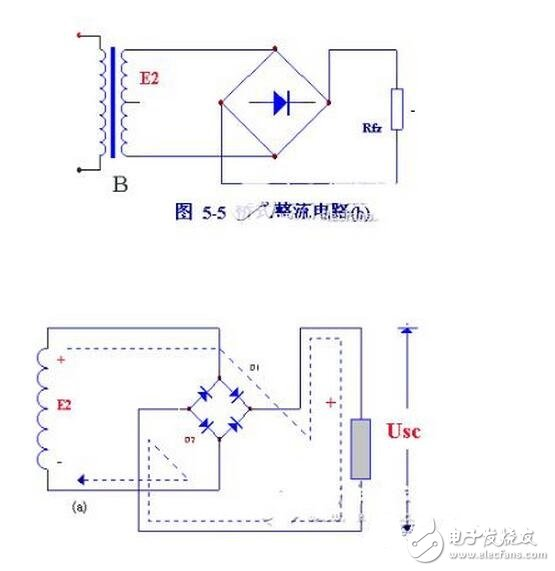Repeating this way, the result is a full-wave rectified voltage at Rfz. The waveform is the same as the full-wave rectified waveform. It is not difficult to see from Figure 5-6 that the reverse voltage of each diode in the bridge circuit is equal to the maximum value of the secondary voltage of the transformer, which is half the size of the full-wave rinsing circuit!

Selection and application of rectifier components

It should be specially pointed out that the diode as a rectifying element should be selected according to different rectification methods and load sizes. . If you choose improperly, you can't work safely, or even burn the tube; or use it too much, causing waste. The parameters listed in Table 5-1 are available for reference when selecting a diode.

"In addition, in the case of high voltage or high current, if the hand does not have a high voltage or a large rectifying rectifier element, the diodes can be used in series or in parallel.

Figure 5-7 shows the diodes in parallel: two diodes in parallel, each sharing half of the total current of the circuit, three diodes in parallel, each sharing one-third of the total current of the circuit. In short, there are several diodes in parallel, "the current flowing through each diode is equal to a fraction of the total current. However, in actual parallel operation," the current passed cannot be evenly divided due to the inconsistent characteristics of the diodes. It will cause some pipes to be overburdened and burned. Therefore, it is necessary to connect a small resistor with the same resistance value in series with each diode so that the current flowing through each parallel diode is nearly uniform. The current sharing resistor R generally uses a resistor of a few tenths of a ohm to several tens of ohms. The larger the current, the smaller R should be selected.

Figures 5-8 show the case where the diodes are connected in series. Obviously under ideal conditions, several tubes are connected in series, and each tube should withstand a reverse voltage equal to a fraction of the total voltage. However, because the reverse resistance of each diode is not the same, it will cause uneven voltage distribution: diodes with large internal resistance may be broken down due to excessive voltage, and thus cause a chain reaction, and the diodes will be broken down one by one. A resistor R connected in parallel on the diode allows uniform voltage distribution. The voltage equalizing resistor should take a resistor whose resistance value is smaller than the reverse resistance of the diode, and the resistance of each resistor should be equal. For resistors with small resistance values, the resistance of each resistor should be equal.Waveform principle analysis of thyristor rectifier circuit:

The ratio of the effective value of a certain voltage (or current) to its average value is called the waveform coefficient. The waveform coefficient in the thyristor rectifier circuit is a significant problem. To illustrate this problem, we first experiment with the thyristor half-wave rectification circuit shown in Figure 1. The model and parameters of each component are for reference only.First turn the R value to the maximum and turn on the power. At this time, the DC voltmeter indicates zero and the lamp does not illuminate. Then slowly reduce the R value, the voltmeter reading gradually increases, and the bulb gradually brightens. We will find that when the DC voltmeter indicates 10 volts, the bulb will reach normal brightness, which means that the power consumption of the bulb has reached the rated power. If the voltage continues to increase, the bulb may burn out. Why is the reading of the voltmeter far below the rated voltage of the bulb of 36 volts, and the power consumption of the bulb has reached the rated power?

The current flowing in the light is a one-way pulsating current, and the voltage across the bulb is a one-way pulsating voltage, and its waveform is shown by the solid line in FIG. The reading of the DC voltmeter is the average of this pulsating voltage, and 刁; is its rms value. Its RMS value is much larger than the average.

According to electrical engineering knowledge, the effective value U of this periodic one-way ripple voltage. Is the square root of the instantaneous value (the root mean square value) of the average value over a period, ie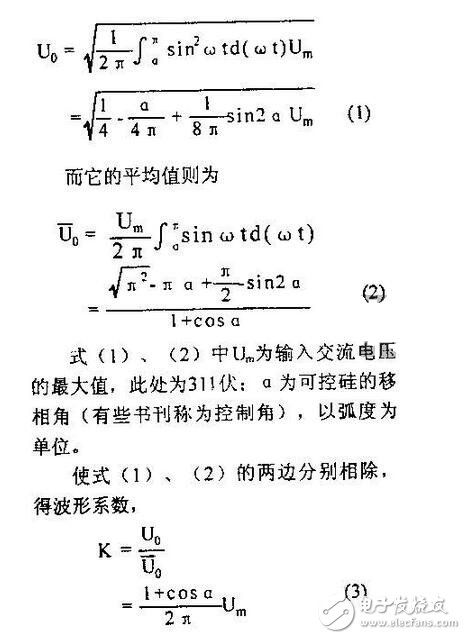Substituting different Q values into equation (3) yields the corresponding K values, as shown in Table 1. It can be seen from Table 1 that when the phase shift angle of the thyristor changes from zero to n, the waveform coefficient K value gradually increases, and the increasing speed becomes faster and faster. Close, at I, the K value will increase sharply (while U and Uo both converge and fall.)

Now let's take a look at the experimental results. According to formula (2), when the DC voltmeter indicates 10 volts, it is U. When 10 volts, CO\$n=-0.7979, the waveform coefficient is K~3.57, Uo~35.7 volts. Uo is quite close to the rated voltage of the bulb, so the bulb reaches normal brightness.

According to the same principle, when G is the same, in the full-wave controlled rectifier circuit of the resistive load, the output ripple voltage (the solid line in the waveform shown in Fig. 3) is 1//2 times the coefficient. In the above calculations, the forward voltage drop when the thyristor is turned on is ignored. The waveform coefficients of the output voltage when other types of rectifier circuits and the load are inductive are not described here.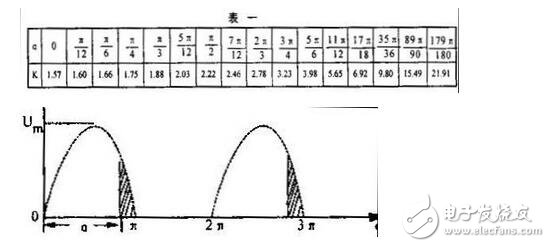From the above analysis, when the thyristor is rectified in a row, the reading of L on the DC voltmeter (or ammeter) is the average 1K value of the output voltage (or current), and the reading cannot be directly substituted into the formula U2 L to calculate. The power consumption on the load, because U is the effective value of the voltage on the load R, ie U=Uo.

If you want to reduce the waveform coefficient so that the output voltage rms value is close to the average value, there are three measures:

(1) Minimize the phase shift angle of the thyristor, such as Q: o, then K = I. 57 (single-phase half-wave): (2) When the rated voltage of the load is much lower than the effective value of the input AC voltage, first step down with a transformer and then rectify; (3) Try to use one-way controlled rectifier or three-phase controllable Rectifier circuit. If the influence of the waveform coefficient is neglected, although the reading of the voltmeter is far from the rated voltage of the load, it is still possible to burn the appliance, resulting in undue loss. This must be noted.In practical applications, for the sake of convenience, we can estimate the waveform coefficients of different output DC voltages according to Table 2, and estimate the effective value of the output voltage. n in Table 2 is the reading U of the DC voltmeter. The ratio of the input AC voltage rms U. That is, 23 (3), the corresponding waveform coefficient K can be obtained. For example, in the circuit shown in FIG. 1, when the DC voltmeter indicates 50 volts, n=50/220~0.23, according to Table 2, the waveform coefficient K at 2.32 and 1.98 can be estimated. between.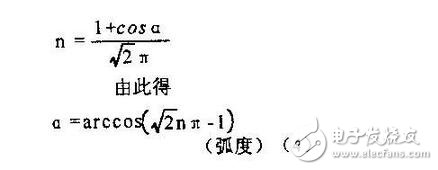For a full-wave controlled rectifier circuit,

According to the same principle, the waveform coefficient K corresponding to different n values (when the thyristor is fully turned on, the maximum value of 0.9 is obtained) can be obtained in the full-wave controlled rectifier circuit.

Communicate with Supplier?Supplier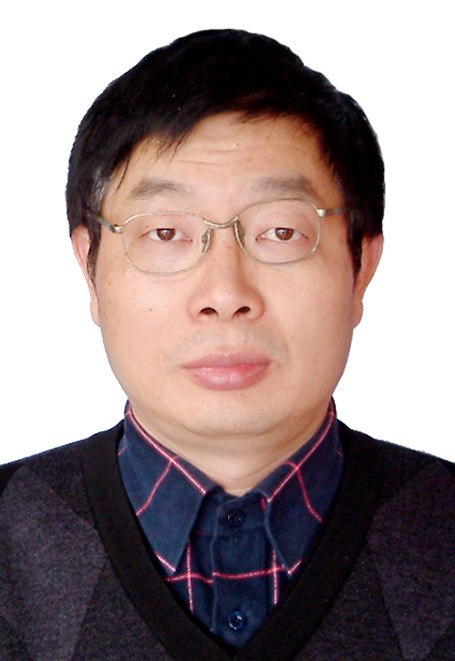Mr. John chang
What can I do for you?